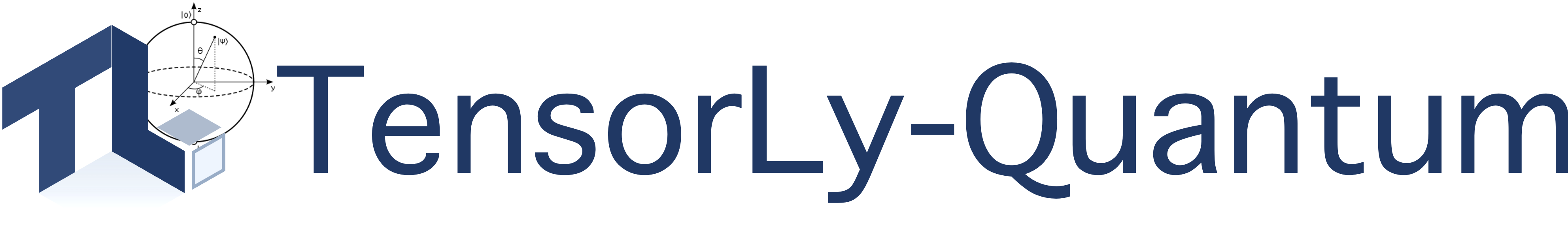### Tensor-Based Quantum Machine Learning

TensorLy-Quantum is a Python library for Tensor-Based Quantum Machine Learning that builds on top of TensorLy and PyTorch.

With TensorLy-Quantum, you can easily:

• Create quantum circuit: .

• Leverage tensor methods: the state vectors are efficiently represented in factorized form as Tensor-Rings (MPS) and the operators as TT-Matrices (MPO)

• Efficient simulation: tensorly-quantum leverages the factorized structure to efficiently perform quantum simulation without ever forming the full, dense operators and state-vectors

• Multi-Basis Encoding: we provide multi-basis encoding out-of-the-box for scalable experimentation

• Solve hard problems: we provide all the tools to solve the MaxCut problem for an unprecendented number of qubits / vertices